# MP Board Class 10th Maths Solutions Chapter 13 Surface Areas and Volumes Ex 13.2

In this article, we will share MP Board Class 10th Maths Book Solutions Chapter 13 Surface Areas and Volumes Ex 13.2 Pdf, These solutions are solved subject experts from the latest edition books.

## MP Board Class 10th Maths Solutions Chapter 13 Surface Areas and Volumes Ex 13.2

Unless stated otherwise, take π = $$\frac{22}{7}$$

Question 1.
A solid is in the shape of a cone standing on a hemisphere with both their radii being equal to 1 cm and the height of the cone is equal to its radius. Find the volume of the solid in terms of π.
Solution:
Here, r = 1 cm and h = 1 cm.
Volume of the conical part = $$\frac{1}{3}$$ πr2h and volume of the hemispherical part = $$\frac{2}{3}$$ πr3h∴ Volume of the solid shape
= $$\frac{1}{3}$$ πr2h + $$\frac{2}{3}$$ πr3h = $$\frac{1}{3}$$ πr2h[h + 2r]
= $$\frac{1}{3}$$ π(1)2 [1 + 2(1)] cm3
= ($$\frac{1}{3}$$ π × 1 × 3) cm3 = π cm3Question 2.
Rachel, an engineering student, was asked to make a model shaped like a cylinder with two cones attached at its two ends by using a thin aluminium sheet. The diameter of the model is 3 cm and its length is 12 cm. If each cone has a height of 2 cm, find the volume of air contained in the model that Rachel made. (Assume the outer and inner dimensions of the model to be nearly the same.)
Solution:
Here, diameter = 3 cm
⇒ Radius (r) = $$\frac{3}{2}$$ cm
Total height = 12 cm
Height of a cone (h1) = 2 cm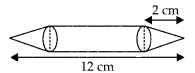∴ Height of both cones = 2 × 2 = 4 cm
⇒ Height of the cylinder (h2) = (12 – 4) cm = 8 cm.
Now, volume of the cylindrical part = πr2h2
Volume of both conical parts = 2[$$\frac{1}{3}$$ πr2h1]
∴ Volume of the whole model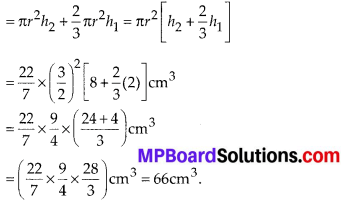Question 3.
A gulab jamun contains sugar syrup up to about 30% of its volume. Find approximately how much syrup would be found in 45 gulab jamuns, each shaped like a cylinder with two hemispherical ends with length 5 cm and diameter 2.8 cm (see figure).Solution:
Since a gulab jamun is like a cylinder with hemispherical ends.
Total height of the gulab jamun = 5 cm.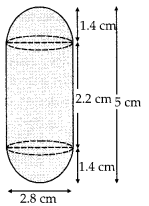Diameter = 2.8 cm
⇒ Radius (r) = 1.4 cm
∴ Length of the cylindrical part (h)
= 5 cm – (1.4 + 1.4) cm = 5 cm – 2.8 cm = 2.2 cm
Now, volume of the cylindrical part = πr2h and volume of both the hemispherical ends
= 2($$\frac{2}{3}$$ πr3) = $$\frac{4}{3}$$ πr3
∴ Volume of a gulab jamun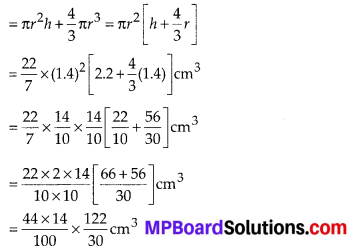Volume of 45 gulab jamuns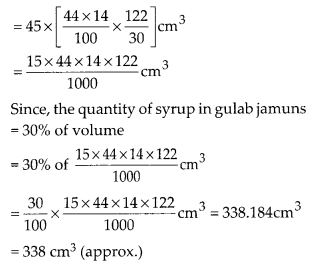Question 4.
A pen stand made up of wood is in the shape of a cuboid with four conical depressions to hold pens. The dimensions of the cuboid are 15 cm by 10 cm by 3.5 cm. The radius of each of the depressions is 0.5 cm and the depth is 1.4 cm. Find the volume of wood in the entire stand (see figure).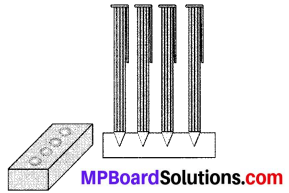Solution:
Dimensions of the cuboid are 15 cm by 10 cm by 3.5 cm.
∴ Volume of the cuboid = 15 × 10 × $$\frac{35}{10}$$ cm3
= 525 cm3
Since, each depression is conical in shape with base radius (r) = 0.5 cm and depth (h) = 1.4 cm
∴ Volume of each depression
= $$\frac{1}{3} \pi r^{2} h=\frac{1}{3} \times \frac{22}{7} \times\left(\frac{5}{10}\right)^{2} \times \frac{14}{10} \mathrm{cm}^{3}=\frac{11}{30} \mathrm{cm}^{3}$$
Since, there are 4 depressions
∴ Total volume of 4 depressions = $$\frac{44}{30}$$ cm3
Now, volume of the wood in entire stand = [Volume of the wooden cuboid] – [Volume of 4 depressions]
= 525cm3 – $$\frac{44}{30}$$ cm3
= $$\frac{15750-44}{30} \mathrm{cm}^{3}=\frac{15706}{30} \mathrm{cm}^{3}$$
= 523.53 cm3Question 5.
A vessel is in the form of an inverted cone. Its height is 8 cm and the radius of its top, which is open, is 5 cm. It is filled with water up to the brim. When lead shots, each of which is a sphere of radius 0.5 cm are dropped into the vessel, one-fourth of the water flows out. Find the number of lead shots dropped in the vessel.
Solution:
Height of the conical vessel (h) = 8 cm
Base radius (R) = 5 cm
Volume of water in conical vessel = $$\frac{1}{3}$$ πR2hThus, the required number of lead shots = 100

Question 6.
A solid iron pole consists of a cylinder of height 220 cm and base diameter 24 cm, which is surmounted by another cylinder of height 60 cm and radius 8 cm. Find the mass of the pole, given that 1 cm3 of iron has approximately 8 g mass. (Use π = 3.14)
Solution:
Height of the big cylinder (h) = 220 cm
Base radius (r) = $$\frac{24}{2}$$ cm = 12cm
∴ Volume of the big cylinder
= πr2h = (π(12)2 × 220) cm3
Also, height of smaller cylinder (h1) = 60 cm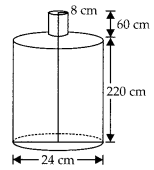Base radius (r1) = 8 cm
∴ Volume of the smaller cylinder πr12h1
= (π(8)2 × 60) cm3
∴ Volume of iron pole = [Volume of big cylinder] + [Volume of the smaller cylinder]
= (π × 220 × 122 × π × 60 × 82) cm3
= 3.14[220 × 12 × 12 + 60 × 8 × 8] cm3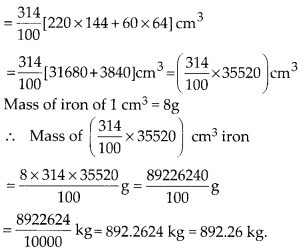Question 7.
A solid consisting of a right circular cone of height 120 cm and radius 60 cm standing on a hemisphere of radius 60 cm is placed upright in a right circular cylinder full of water such that it touches the bottom. Find the volume of water left in the cylinder, if the radius of the cylinder is 60 cm and its height is 180 cm.
Solution:
Height of the conical part = 120 cm
Base radius of the conical part = 60 cm
Volume of the conical part
= $$\frac{1}{3}$$ πr2h = [$$\frac{1}{3} \times \frac{22}{7}$$ × (60)2 × 120] cm3
Radius of the hemispherical part = 60 cm.Question 8.
A spherical glass vessel has a cylindrical neck 8 cm long, 2 cm in diameter; the diameter of the spherical part is 8.5 cm. By measuring the amount of water it holds, a child finds its volume to be 345 cm3. Check whether she is correct, taking the above as the inside measurements, and π = 3.14.
Solution:
Volume of the cylindrical part = πr2h
= (3.14 × 12 × 8) cm3 = $$\frac{314}{100}$$ × 8 cm3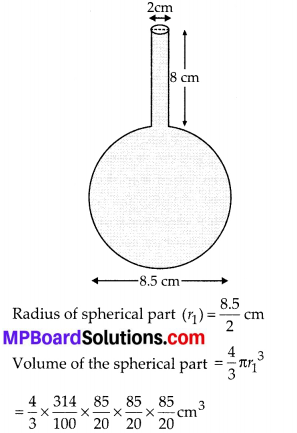∴ Total volume of the glass vessel = [Volume of cylindrical part] + [Volume of spherical part]⇒ Volume of water in the vessel = 346.51 cm3
Since the child finds the volume as 345 cm3
∴ The child’s answer is not correct.
The correct answer is 346.51 cm3.